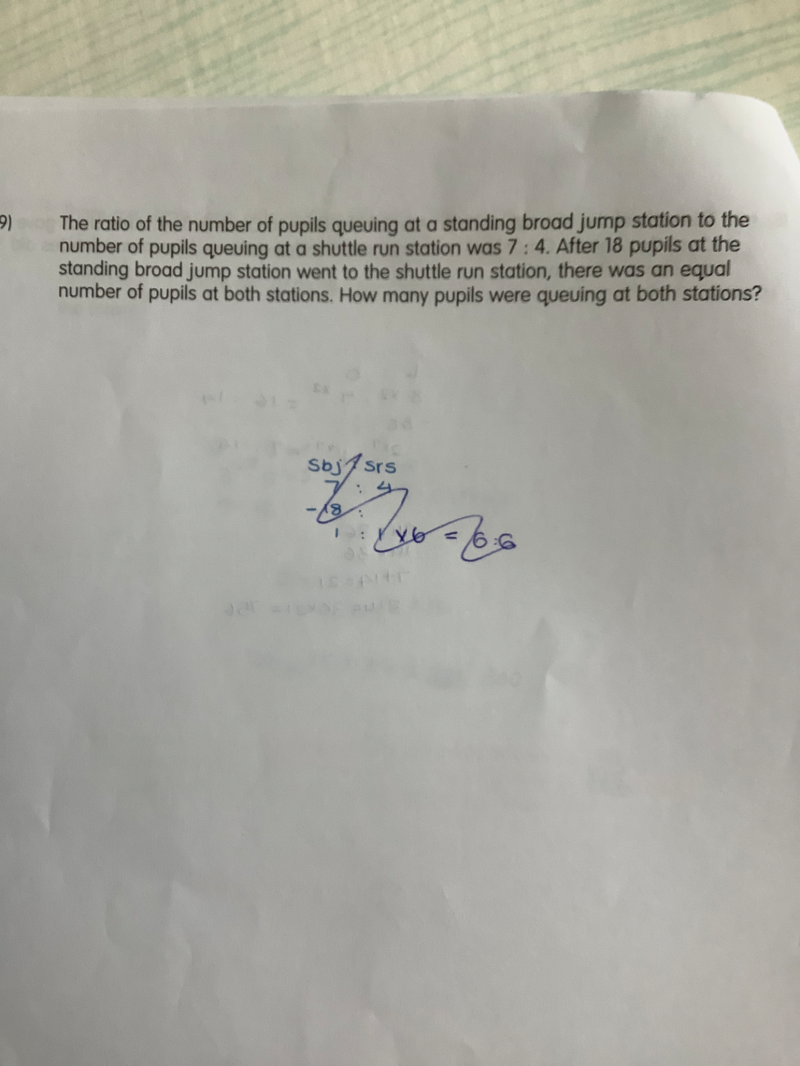# QuestionC

hi, need help with this maths problem. Thank you!

Queuing At first                         7u                                           4u

18 from SBJ to SR                  -18                                           +18

Then the queues are equal, this implies

7u-18 = 4u+18

3u = 36

u =12

Hence queuing at SBJ  was 7u = 84 and queuing at SR was 4u = 48

At the end, both stations have  (84+48) = 132 pupils with each 66 pupils.

0 Replies 1 Like

```Thinking:
Ratio is Jump:Run = 7:4. So we can use 7u:4u, a total of 11u.
After some movement they become equal, i.e. divisible by 2.
But 11 is not divisible by 2, so let's scale up by 2 instead.
We use Jump:Run = 14u:8u with a total of 22u (so 22u/2 = 11u).

Working:
JUMP      RUN
BEFORE       14u       8u    (total of 22u students)
AFTER        11u      11u    (3u students moved)

So 3u = 18 and u = 6.

Thus at the start, there are 14 x 6 = 84 students in standing
board jump queue and 8 x 6 = 48 students in shuttle run queue.
At the end, there are 66 students in both queues.

Checking:```
```            JUMP      RUN
BEFORE       84        48
AFTER     66=84-18  66=48+18 (18 students moved)```